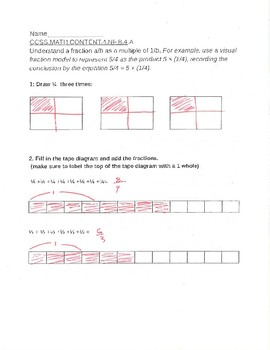# Whole number multiply by a unit fraction formative assessmentSubject
Resource Type
File Type

PDF

(178 KB)
Standards
• Product Description
• StandardsNEW

This is a 1,2,3,4 formative assessment for CCSS.MATH.CONTENT.4.NF.B.4.A

Understand a fraction a/b as a multiple of 1/b. For example, use a visual fraction model to represent 5/4 as the product 5 × (1/4), recording the conclusion by the equation 5/4 = 5 × (1/4).

Understand a fraction 𝘢/𝘣 as a multiple of 1/𝘣. For example, use a visual fraction model to represent 5/4 as the product 5 × (1/4), recording the conclusion by the equation 5/4 = 5 × (1/4).
Total Pages
N/A
N/A
Teaching Duration
N/A
Report this Resource to TpT
Reported resources will be reviewed by our team. Report this resource to let us know if this resource violates TpT’s content guidelines.